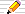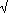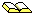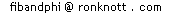Theicon means there is a Things to do investigation at the end of the section.

## What is a "Fibonacci Forgery"?

Sometimes we find a series of numbers which looks as if it is the Fibonacci series, but, when we look at bit further, we discover it isn't! These are the Fibonacci Forgeries! A good forgery will have many terms that are the same as the Fibonacci numbers. This page is about those forgeries. So beware if you think you have found a new disguise for the Fibonacci numbers. It may indeed be a new formulation but then it could possibly be a counterfeit! Only a mathematical or logical proof can guarantee that your formula is indeed exactly the Fibonacci numbers.

# A simpler formula using Phi?

On the Formula for the FIbonacci Numbers page, we first saw Binet's Formula for Fib(n):
 Fib(n) = Phin – (–Phi)–n = Phin – (–phi)n55

We also showed how it could be simplified to
 Fib(n) = roundPhin5
But Tim Crocker and Robert Kybird have found a simpler formula:
Fib(n) = round( Phin + 1/3 )
Here it is tabulated:
nPhi(n+1/3)rounded
0 1.17398 1
1 1.89955 2
2 3.07353 3
3 4.97308 5
4 8.04661 8
5 13.01969 13
6 21.06631 21
7 34.08600 34
8 55.15230 55
9 89.23830 89
10 144.39061 144
The rounded values are certainly the first numbers of the Fibonacci series -
but it is a counterfeit:-

The next row of the table is
Phi11+1/3 = 233.6289136 which rounds to 234.

But the next Fibonacci number, being the sum of the last two in the list above, should be 89 + 144 or 233 and not 234!

Could we rescue the formula by using floor instead of round?
[ The floor function rounds down by taking a non-integer value to the next integer below it. For positive real numbers, this just means remove the decimal part. ]
This would give 283 for n=11 in the table above. Looking at the rest of the table, you will see that it now alters some previously correct values too. For instance, when n is 3, Phi3+1/3 = 4.97308 and the floor of this number is 4. But the Fibonacci number is Fib(3)=5 not 4.If you like, you can skip this section marked by the green line on the left.
It explains why this is a good forgery.

### Why is it a close fit?

The reason it almost works is that we can express Phin/5 as Phin+c for some number c.

To do this we will need logs to base Phi. We met logs previously when finding the length of a number .

The LOG button on your calculator will do the opposite of the xy button, which raises one number (the base) to the power of another (the exponent): baseexponent.
The LOG button will find the exponent for you.
However, the LOG button uses a fixed base. This is 10 for LOG button and e for the LN button.
So, for instance,
LOG 1000 will display 3 because 103 is 1000. and
LN 1000 = 6.9077.. because e6.9077..=1000.

For instance the log of 8 to base 2 is 3 since 23 is 8.
Log-to-base-b of x means "What power of the base (b) will give the number x?".
It is fairly easy to show that we can get away with just one log button on a calculator and with it we can find logs to any base.

First, we note that to write "log to base b" we will use the mathematical notation logb. So LOG(x) means log10(x) and LN(x) means lobe(x).
The formula is:

 logb(x) = LOG(x)LOG(b)
We could just as easily replaced LOG by LN too.
Let's check that "The log-to-base-2 of 8 is 3.":
 log2(8) = LOG(2) = 0.3010 = 3.0LOG(8)0.9030
Now we are ready to tackle the question we started with - how to write Phin/5 as Phin+c = Phin Phic for some number c. We need c which is 1/5 as an exponent of Phi, that is, c=logPhi(1/5).
Here are some rules of logs that we will need:
log(1) = 0 (since b0 = 1 for all bases b
log( xy ) = log(x) + log(y) for all bases log( xz ) = z log(x)
Since5 = 51/2 and 1/5 = 5-1/2
then logPhi(1/5) = logPhi(5-1/2) = -logPhi(5)/2
Using the formula above for logPhi(x), we have
logPhi(5) = LOG(5)/LOG(1.61803..) = 0.69897/0.20899 = 3.34455
so logPhi(5)/2 is 3.34455/2=1.67227
However, the formula that Tim and Robert found is not strictly a formula for Fib(n), but for Fib(n+2) as we can see in the table on the right here:
nPhi(n+1/3)roundedFib(n)
0 1.17398 10
1 1.89955 21
2 3.07353 31
3 4.97308 52
4 8.04661 83
5 13.01969 135
6 21.06631 218
7 34.08600 3413
8 55.15230 5521
9 89.23830 8934
10 144.39061 14455
So their formula:
round( Phin+1/3 ) is for Fib(n+2)
and we know that
Fib(n+2) = round(Phin+2/5).
We have just seen that5 is Phi1.67227, so
 Fib(n+2) = round( Phin+2/Phi1.67227 ) = round( Phin+2-1.67227 ) = round( Phin+0.32773 )
More accurately still, the exponent is 0·32772 40618 15445 25382 96808 73605 56344 60715 00579 857919 9... .

So the true value of 0.32772 is very near 1/3 which is why 1/3 works so well.
If we calculate the values of Phin+0.33, this agrees with Fib(n+1) up to n=12 (so it is already more accurate than Phin+1/3) and Phin+0.3277 is better still, being correct up to n=21 whereas 0.327724 is correct up right up to n=34.

Stop skipping now!
Here comes the next Fibonacci Forgery...

## A formula using e?

Someone suggests to you that the following is another formula for the Fibonacci numbers - is it?
G(n) = ceiling( e(n-2)/2 )) = ceiling( (e)n-2)
where the "ceiling" function means "the next integer above" (eg: ceiling(2·1)=3 and ceiling(2·9)=3 also). This is a remarkable formula since we get:
 n: G(n): 1 2 3 4 5 6 7 8 1 1 2 3 5 8 13 21
but it is, in fact, a forgery!
ReferencesR K Guy in The Second Strong Law of Small Numbers in The Mathematics Magazine (1990), Vol 63, pages 3-20, example 41 adapts an inequality of Larry Hoehn's to get this surprising coincidence.

####Things to do1. How far does G(n) go before we no longer get the successive numbers of the Fibonacci series appearing?

## A Polynomial formula for Fib(n)?

A polynomial in x,P(x), is a sum of various powers of x and their (positive or negative) multiples.
The highest power of x which occurs is called the degree of the polynomial. A polynomial of degree 1 is called linear;
a polynomial of degree 2 is called quadratic;
a polynomial of degree 3 is called cubic; etc.
If the polynomial has an infinite number of powers of x, it is called a power series.

### A simple polynomial

Here is a simple example of a linear polynomial P(x) which gives the first 3 values of the Fibonacci series, that is, P(1)=1, P(2)=2 and P(3)=3 :
```      P(x) = x
```
but that doesn't give P(4)=5, which is what we want for the real Fibonacci series, so this P(x) is a Fibonacci forgery.

### Another polynomial

Can you find a polynomial Q(x) which gives Q(1)=1, Q(2)=2, Q(3)=3 and Q(4)=5? Here is a cubic polynomial which does that:
Q(x)= ( x3 - 6 x2 + 17 x - 6 )/6
but Q(5) is... 9 whereas Fib(5)=8, so this is another, but better, forgery! How about...

### And another!

Here's an example of a "Fibonacci Forgery" polynomial p(x) for which p(1)=1, p(2)=2, p(3)=3, p(4)=5 and p(6)=8 so that its first 6 values look like the Fibonacci series. However, here, p(7)=a and "a" can be any value you choose!
p(x) = [ (a-11) x5 + (160-15a) x4 + (85 a-865) x3 + (2180-225 a) x2
+ (274 a - 2424) x - 120 a + 1080 ] / 120;
Or, if you want both 1's at the beginning of your series, then the following version has 1, 1, 2, 3, 5, 8 and then "a" as its first 7 values:
p(x) = [ (a-8) x6 + (150-21 a) x5 + (175 a-1070) x4
+ (3630-735 a) x3 + (1624 a - 5762) x2
+ (3780 - 1764 a) x + 720 a ] / 720;

####Things to do1. It looks like, given the 4 values P(1),P(2),P(3) and P(4) we can find a degree 3 polynomial for P (ie one whose highest power of x is 3); and given 6 values a degree 5 polynomial and given 7 values a degree 6 polynomial. However, the first 3 values were fitted to a linear (degree 1) polynomial.
Is it always true that given N values for P(1) to P(N) then we can find a polynomial P which has degree at most N-1? [Consult your teacher or maths library at college.] If so, how do we calculate the polynomial?

In the December issue of The Mathematics Teacher (ISSN 0025-5769) a letter from Deborah Freedman a student at Framingham High School, MA, USA, conjectured that the following series was the Fibonacci numbers.
In how many ways can n segments of equal length be connected in a plane if the beginning of one segment is to be connected to the end of the previous segment at a right-angle? Congruent configurations are to be counted as one.
From the examples given, we can clear up a few questions left by this definition.
By congruent, she means that a shape can be rotated or reflected and it still counts as the same shape. So for 3 links, there are just two "shapes":
```
oooo   oooo      o         o          o  o   oooo  oooo   oooo
o         o      o         o          o  o = o   = o  o =    o
oooo   =     oooo = oooo = oooo    and   oooo   oooo  o  o   oooo
o   o
o   o
```
The sequences are not to overlap, that is, a later segment cannot lie on top of an earlier one, so that each diagram of n links has exactly n straight line segments in it. Links can cross over (at the ends where they join others) and we can have "squares" in our link chains for example:
```    ooooooo      oooo            ooooooo           ooooooo
o  o  or  o  o      or    o  o  o  but not  o  o  o
oooo      ooooooo         ooooooo           ooooooo
o  o            o  o           o  o  o
oooo            oooo           ooooooo
```
since the last shape cannot be made from 12 links in a single chain (in other words, it cannot be drawn in one go, without taking your pen off the page and without going over any line twice).
Deborah's table of small solutions is:
```number of                                                    number of
segments                  configurations                       ways
-----------------------------------------------------------------------
1                             ooo                              1
-----------------------------------------------------------------------
2                             ooo
o                                1
o
-----------------------------------------------------------------------
3                          ooo         o o
o           o o                     2
ooo           ooo
-----------------------------------------------------------------------
4                      ooo        ooo o        ooo
o            o o        o o             3
ooo            ooo        ooo
o
o
-----------------------------------------------------------------------
5                   ooo   o      ooo o    ooo ooo      o
o     o      o o o      o o        o
ooo     ooo o  o ooo      ooo      ooo       5
o         o o                      o o
ooo         ooo                      ooo
-----------------------------------------------------------------------
6     ooo  ooo     ooo ooo  o        ooo    ooo o  ooo   ooo
o      o       o o o  o        o      o o o  o o   o o
ooo      ooo o   ooo o  ooo ooo  ooo o  ooooo  ooo   ooooo
o          o o            o o      o o           o     o
ooo          ooo            ooo      ooo           ooo   o   8
o
o
-----------------------------------------------------------------------
7      ooo  o                 ooo           ooo      o  o     o
o    o                 o o           o        o  o     o
ooo    ooo      o ooo o  o ooo o   ooooo  ooo ooo  ooo ooo
o        o      o o o o      o o   o o    o o o      o o
ooo        ooo o  ooo ooo      ooo   ooo    o ooo      ooo
o            o o
ooo            ooo

ooo  ooo       ooo o      o o
o  o         o o o      o o                     13
ooo ooo     ooooo  ooo ooo   ooooo    ooooo   ooooo
o o o       o o      o o       o      o o     o o o
ooooo       ooo      ooo       o      ooo     ooooo
-----------------------------------------------------------------------
```
[With thanks to Jeff Myers, Granville High School, OH, USA for part of this table and for pointing out this problem in The Mathematics Teacher.]

How many shapes are there with 7 links? Try it and you'll find the number of 7-link shapes is 15. In her listing in the The Mathematics Teacher she only gave 13, and missed the following two shapes with 7 links:

```       ooo
o o
ooo ooo        ooo ooo
o              o o o
ooo            ooo ooo
```
These were generated by a computer program (in Prolog), so, if my programming is correct, there aren't any more shapes missing. The program also showed that The number of 8-link shapes is 23 and this should be 21 if the Fibonacci numbers were the correct series. There are 43 9-link shapes and again this should be 34 if the Fibonacci numbers were involved.

So - Deborah's conjecture looks interesting - that there are Fib(n) shapes that can be made from n links at right-angles with no overlapping and allowing for rotations and reflections, but it is another forgery!

[There is an online WWW page for The Mathematics Teacher.]

Mark Lewis and John Moore have a page on Fibonacci Forgeries which is a summary of a Scientific American article of May 1995 by Ian Stewart on series that look as if they are the Fibonacci numbers to start with, but which turn out not to be.

## ReferencesRichard K Guy in The Second Strong Law of Small Numbers in The Mathematics Magazine (1990), Vol 63, pages 3-20
mentions the Pennies Puzzle 1 and Pennies Puzzle 2 on the Harder Fibonacci Puzzles page and that only one of them is truly Fibonacci.

This fun paper also has several other Fibonacci Forgeries including ones on partitions of n, rooted trees with one label, the number of disconnected graphs on n+1 vertices and the number of connected graphs on n+2 vertices which have just one cycle.

There are many other forgeries in the paper to do with primes, Catalan numbers, binomial and trinomial numbers, mixing some genuine examples with the forgeries. His whole point is that There are not enough small numbers to meet the many demands made of them and so we are bound to be fooled with small examples of a problem if we are not careful! Also see..The Strong Law of Small Numbers Richard K Guy in The American Mathematical Monthly, Vol 95, 1988, pages 697-712.© 1996-2006 Dr Ron Knottupdated 16 April 2006